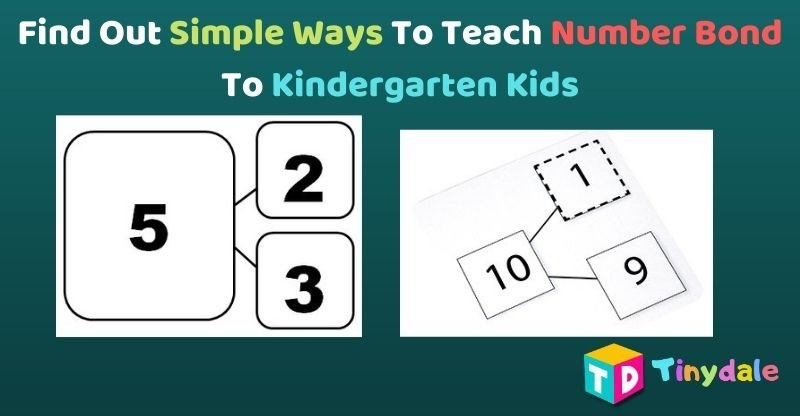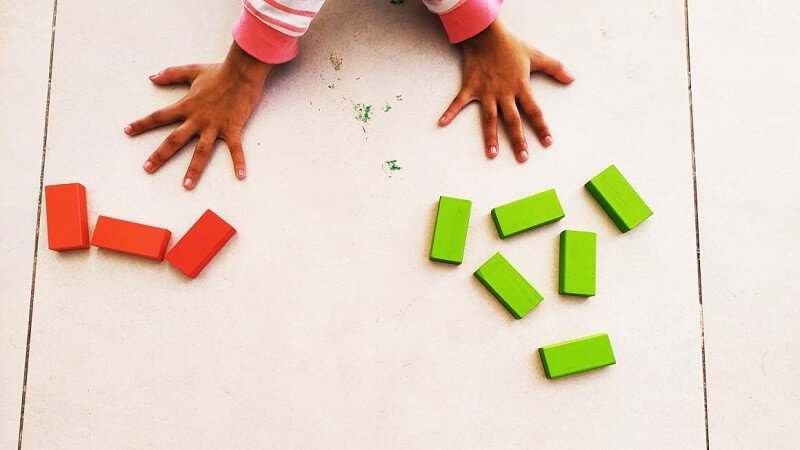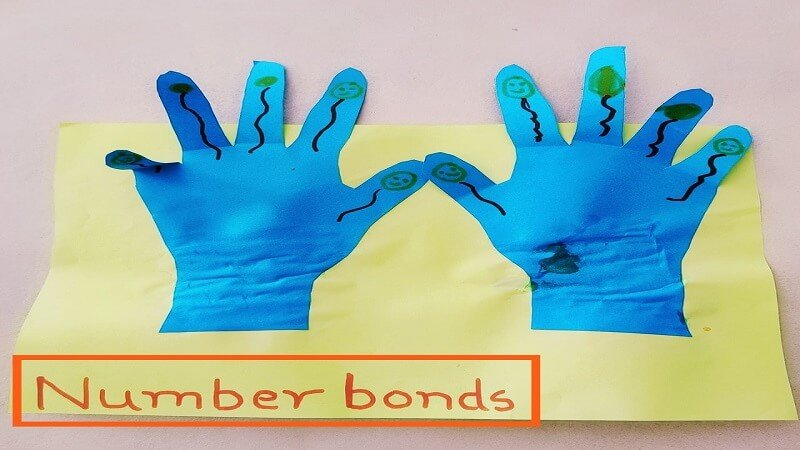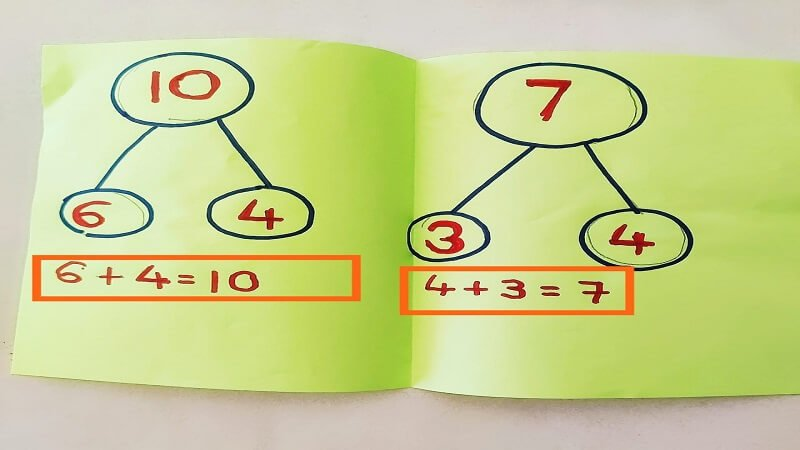Tuesday, September 14, 2021
Learning

# 5+ ways to teach Number bond to 10 for kids – tinydaleWhat are the number bonds to 10? Number Bond is a simple addition of two numbers, which makes a sum a big number. Simply, It is a pair of numbers which by adding, make the specific number. Basically, they are number pairs. For kindergarten kids, we use simple drawings to make them understand the concept of number bonds to 10.

Like for example, Numbers Bond to 10 are as follows:

• 9+1=10
• 8+2=10
• 7+3=10
• 6+4=10
• 5+5=10

Number Bond to 5 are as follows:

• 2+3=5
• 1+4=5

In the above example, We saw some pairs which add together to make 10 and 5. These pairs in the above example are called number bonds. Basically, the Number bond is the pair between a number and the parts that form it.

Before that please don’t forget to

## Simple Ways To Practice Number Bond to 10 with kids

Some of the simple ways to build the concept of number bond in kindergarten kids by following ways:

## 1. Wooden Blocks for kids

• The simplest way is by the use of objects/things. The objects can be placed in front of kids. Let them sort out the objects. Like for example, If a kid has 6 green wooden blocks and 3 red wooden blocks. Tell him to sort out and separate based on color. Then let him add using number bonds and find out the answer.You can tell them to make the number bond of 5 using these colorful wooden blocks.## 2. Number bond activities

• Another way is to take a colored A3 size paper. Now trace both of your hands with a palm and cut them properly. Now stick both the cutouts on plain colored paper. Let your kid draw some freehand on each finger. Now let him interact with the moving fingers. Let him count by folding the fingers required. Like for example, when we add 5 fingers plus 5 fingers, it makes 10. In this way, let him explore to make a Number bond till 10 using this Hand activity for a child.## 3. Bond Number format

• Next, you can make them understand the concept shown in the picture below. Make 3 circles; the big circle should contain the addition of the two numbers. The smaller circle contains the number which we add together to make a big number. For example, in the image shown below, we will put 6 and 4 in small circles as when we add them, we get 10, which cokes in a big circle.## 4. Practice and Practise

You can make them practice in their respective notebook. They can understand the concept of number bonds by performing simple additions.

## 5.  Maths Objects

• In daily routine, You can make them understand using different objects. Like taking 3 balls and adding 2 more balls, we get 5 balls.## Review And Closing

So, This is all about the number bond to 10. I practice all this with my kindergarten kid. These hands-on simple ideas make her understand the concept very well. Gradually, You can introduce the concept of whole and part relationships as in whole numbers and their parts. This is how my child and I love to make learning fun. These are the top 5 hands-on activities initiated by me for my children to learn math. Please feel free to share your experiences with math kindergarten. You can get more of our simple lesson plans in the learning category. Do not forget to share your stories with us on mytinydale@gmail.com, If you would like to get featured on our platform.